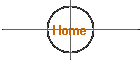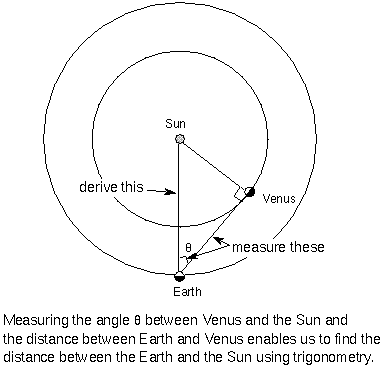### How Far Away is the Sun?

Astronomers determined the size and shape of our galaxy and measured distances to other stars using the “parallax method” (and eventually other methods using the star’s light spectrum).  Unfortunately, these methods do little to help us find out the exact distance to our very own star, the Sun.  Surprisingly, to get the distance to the sun, astronomers first had to determine the exact distance to Venus!

With the invention of radar, scientists were able to measure the distance to Venus very precisely.  By timing how long it took the radar beam to travel at the speed of light to Venus and back, the total distance to the object can be determined from the equation:

Distance = (Speed of Light) X (Total Time)/2

The reason the total time is divided by two is to get just the distance from the Earth to the object.Earth-Sun Distance = (Earth-Venus Distance) / (Cos q)

Using trigonometry, astronomers now know that the “Astronomical Unit” = 92,955,820.5 miles (149,597,892 kilometers).  This incredible degree of accuracy is possible because the speed of light is known very precisely and extremely accurate clocks are used to time the radar reflection.  Radar can’t be used to determine the distance to the Sun directly because the Sun doesn’t have a solid surface to reflect the radar efficiently.

Ref: Strobel’s Astronomy Notes, May 2001.

11/2011

 10/01/2017